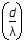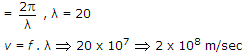# Electronics and Communication Engineering - Electromagnetic Field Theory

21.

Velocity of the wave when it passes from air into sphere

 A. decreases B. increases C. same D. no change

Explanation:

No answer description available for this question. Let us discuss.

22.

The ratio of the directivity of an end fire antenna to that of a broadside antenna is

 A. 2 B. 3 C. 4 D. 5

Explanation:

In Broadside, D = 2nIn End fire D = 4n.

23.

If the electric field intensity associated with a uniform plane electromagnetic wave travelling in a perfect dielectric medium is given by
E(z, t) = 10 cos (2p x 107t - 0.1 pz) volt/metre, then the velocity of the travelling wave is

 A. 3.0 x 108 m/sec B. 2 x 108 m/sec C. 6.28 x 107 m/sec D. 2 x 107 m/sec

Explanation:

E(z, t) = 10 cos (2p x 107t - 0.1 pz)

f = 107 Hz, β = 0.1p24.

The absorption of radio waves by atmosphere depends on

 A. their frequency B. their distance from the transmitter C. the polarization of atmosphere D. the polarization of the waves

Explanation:

No answer description available for this question. Let us discuss.

25.

For transmission-line matching over range of frequencies, it is best to use a

 A. single stub of adjustable position B. double stub C. broadband directional coupler D. balun